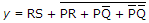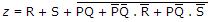# Electronics and Communication Engineering - Digital Electronics - Discussion

29.

If the functions w, x, y, z are as follows.
w = R + PQ + RS ,
x = PQR S + PQR S + PQ R SThen

 [A]. w = z x = z [B]. w = z, x = y [C]. w = y [D]. w = y = z

Explanation:

Use k-map, then it will be easy.

 Meenu Singh said: (Apr 2, 2015) Can anyone please help me solving it using k map, since the expression of 'y' and 'z' involves both min-terms and max-terms?

 Neelima said: (Aug 22, 2015) Please can any one explain this?

 Sharath said: (Jan 4, 2017) Can anyone explain clearly?

 Mythily said: (Sep 16, 2017) Can anyone please explain the answer?

 Prans said: (Nov 30, 2017) The answer a is incorrect. Now, for all values of pqrs (0000 to 1111) must satisfy the conditions. For the example 1010, x evaluated to 0. While z is evaluated to 1. So the answer is wrong.

 Swarag K said: (Apr 16, 2018) How to solve it by using k map? Please explain.

 Pandu said: (Jul 3, 2018) Solve this by assuming the values.

 Md Ramish said: (Aug 2, 2018) @Prans. But x and z are equal.

 Pravallika said: (Aug 28, 2018) What is the difference between p'q'r' and p'q'.r'?

 Mishal said: (Dec 3, 2018) w=z and x=z (bar) is the right answer.

 Anuj Kumar said: (Dec 15, 2018) Can anyone solve this for me with the help of K- map?

 Hema said: (Dec 22, 2018) Hi, I am not getting this, Can anyone slove for me?

 Swathi said: (Jul 19, 2019) Please give me the clear explanation.

 Surya said: (Aug 3, 2019) X=PQR'S' +P'Q'R'S' +PQ'R'S'. If x is equal to this term, the answer will be w=z x=z'.

 Veena Reddy said: (Jun 7, 2022) Can anyone please solve this in detail?